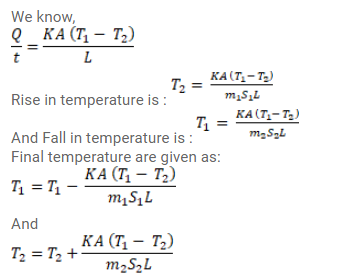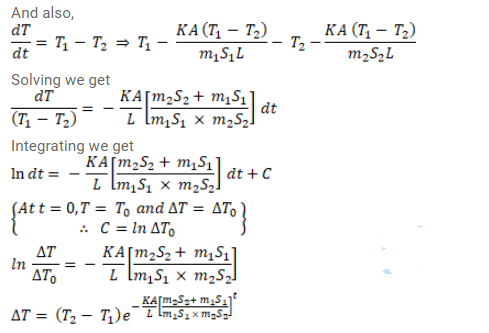# Two bodies of masses m1 and m2 specific heat capacities s1 and s2Question:

Two bodies of masses $m_{1}$ and $m_{2}$ and specific heat capacities $s_{1}$ and $s_{2}$ are connected by a rod of thermally insulated. At time $t=0$, the temperature of the first body is $T_{1}$ and the temperature of the second body is $T_{2}\left(T_{2}>T_{1}\right)$ Find the temperature difference between the two bodies at time $t$.

Solution: